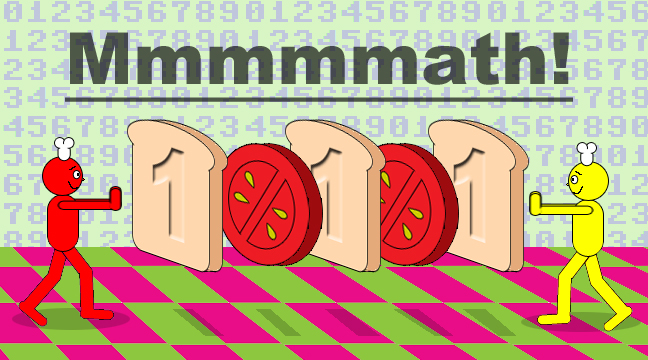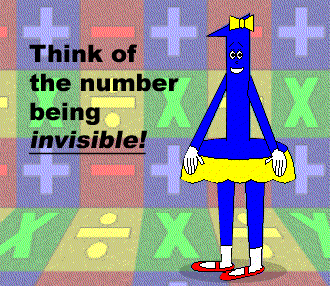# Number Sandwiches

## In reference to the previous math trick, this one is about a summation formula that creates number sandwiches!Remember that the 1's are the bread slices & the zeroes are the toppings!d = The number of decks

m = The number of zeroes in each deck + 1

n = The chosen power of 10

S = The number sandwich

(S is the dependent variable)

## Examples:## S = 100,001 in this example.## S = 101,010,101 in this example.## S = 1,000,100,010,001 in this example.## Have you noticed there are no zeroes in the final example? If the variable m = 1, then it disappears from the formula so that n is multiplied by 1! And since 1 is the number of multiplicative identity, the quantity remains unchanged! People don't bother printing the number 1 next to a variable because of this!## Since 0 = 1 - 1 & m = 1 in this case, there are no toppings(zeroes) in this number sandwich! (Wait a minute! If there are no toppings between bread slices, that doesn't count as a sandwich, does it? Well, at least it's a stack of bread slices.)